Next: The limit command Up: Preliminaries about Maple Previous: The Maple worksheet

Subsections

# Assignments, Functions and Constants

Let us discuss the syntax of some basic Maple commands, as well as some functions and constants that Maple knows.

## Assignment statements

The assignment is the most basic and used of Maple statements. It allows you to give names to expressions you want the computer to remember for later use. It has the colon-equals format  name := expression. The thing on the left side of := is a name, i.e. a sequence of letters and digits that begins with a letter. Certain names must be avoided because Maple uses them for special purposes (see section 4.4):
>
a:=3; b:=5; c:=22/7;Now you can use a, b and c as though they are numbers. Thus, we have

>
a+b;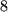>
c*a;>
a-b^2;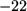## Variables and subsequent assignments: unassignments

If you type a variable name and a semicolon, Maple will tell you what the variable stands for:
>
c;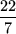>
x;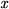If you specify y as an expression involving x and then assign a particular value to x, this value will get substituted into y:

>
y:=sqrt(x+3);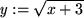>
x:=sin(w);>
y;If x gets changed, then y will also change. Maple remembers the original definition of y:

>
x:=cos(u);>
y;Notice that Maple evaluates x before telling you what the variable y stands for now. To undo this kind of thing, you can unassign variables. To do this for x execute:

>
x:='x';
y;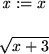We can also tell Maple to forget entirely all assignments made so far using the restart command.

>
restart;
x; y; z;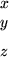Surrounding an expression in single quotes1.10tells Maple to delay evaluation of it. This is why x:='x' unassigns x-- x is assigned to mean just x without any evaluation. Note that stopping evaluation does not stop simplification:

>
'x + 2 + 5';## Functions known to Maple. How to define your own functions

Maple has all the standard mathematical functions in its library. You can make use of them by their appropriate name. We list some of these.
• Trig and inverse trig functions: sin, cos, tan, cot, sec, csc, arcsin, arccos, arctan, arccot, arcsec, arccsc.
• Exponential and logarithm: Maple uses exp(x) for ex and either log(x) or ln(x) for the natural logarithm lnx. Logarithm in other bases are also available. For example, log(x) is log10x.
• Hyperbolic functions: sinh, cosh, etc.
• Square roots and absolute values: sqrt(x) and abs(x).
A much more complete list can be found by asking Maple for help on the initially known functions; ?inifcns does this.

Of course, you will find yourself in a situation where you would like to define your own functions. Suppose you are analyzing the expression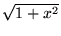, plugging several values into it and calculating the result. Maple does not have a built-in function to do this, but you can define one yourself:

>
f:=x->sqrt(1+x^2);Carefully notice the syntax. f is the name of the function and is written to the right side of :=. The function f sends the variable x to the expression; this is indicated by the arrow. The arrow -> is made with the minus and greater-than keys.

After such an assignment, you can use the name f in the same way you use other names of functions known to Maple, e.g. exp or sin:

>
f(0); f(1); f(-1); f(3*x+1);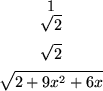We are so accustomed to the notation f (x) to refer to a function that we might accidentally use it in Maple. Never try to define a function with an assignment like

>
f(x):=sqrt(x-2);It seemed like this worked, but in fact, the resulting f(x) does not work as expected. You will not be able to substitute into it or do anything you normally do with functions.1.11

Maple uses parentheses ( ) to group expressions together in a convenient form as in

>
2*(x+4)^3;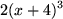or to delimit the arguments of a function. In mathematics, we sometimes cheat and write sinx instead of sin(x). In Maple, this is not permissible: you must always write sin(x). Nested parentheses are permitted:

>
f(x);>
sin(sqrt(x+2)+ln(abs(x)));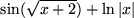## Special constants and reserved names

Maple knows several constants. To find them out, execute the command

>
constants;The names of these constants are false, gamma, infinity, true, Catalan, FAIL and Pi, respectively. Be careful! Maple is case sensitive. Therefore pi is not the same as Pi. For example,

>
cos(Pi/4);>
cos(pi/4);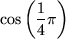Maple also uses I to represent the complex number. Earlier versions used E for exp(1); this is no longer the case. If you'd like to add E as a constant, you can do the following:

>
E:=exp(1);
protect(E);
constants := constants, E;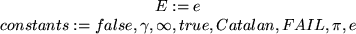While protecting your new constant E will stop you from accidentally redefining it, adding E to the list of known constants doesn't really affect things very much.

There are many commands and special objects in Maple that have preassigned names. In creating your own expressions and setting the name of your own variables, you cannot use any of the following 30 keywords:


and 		 by 		 do 		 done 		   elif 		 else 		   end 		    fi 		 for 		 from
if 		 in 		 intersect 		  local 		 minus 		   mod 		 not 		   od 		 option 		 options
or 		 proc 		  quit 		   read 		 save 		  stop 		 then 		 to 		 union 		  while

You can check this list for yourself using the help system by giving the command ?keywords.

Maple also starts with a large number of predefined functions and constants. Many of these can be listed with inifcns and ininames. If you try to use one by mistake, you will receive an error:

>
gamma:=x^2+5;If you insist, you can use unprotect to allow you to assign to this name. But don't do this unless you know what you are doing; this changes the meaning of this name even when it is used internally, and can have unexpected consequences.

#### Footnotes

... quotes1.10
Be careful! Most keyboards have three different kinds of quotation marks, and they each mean something different to Maple. The single quote (') delays evaluation, the double quote (") indicates a string, and the backquote () indicates a name (similar to a string).
... functions.1.11
Maple uses this notation for a `remember table''-- you can use it to set specific values for the function. For example, if we first define f by f:=x->sin(x)/x, then asking for Maple for f(0) will give an error, since it is not a permissible value for the function. However, we can define f (0) = 1 with the command f(0):=1; after this, f(0) will be 1, while f (x) = sin(x)/x for all other values of x.Next: The limit command Up: Preliminaries about Maple Previous: The Maple worksheet

Translated from LaTeX by Scott Sutherland
2002-08-29